# TRIGONOMETRIC RATIOS UPTO TRANSFORMATIONS, 2

Mathematics Intermediate 1st year mathematics 1A and 1B solutions for some problems. These solutions are very easy to understand.

Inter first year 1A : Functions, mathematical induction, addition of vectors, trigonometric ratios upto transformations, trigonometric equations, hyperbolic functions, inverse trigonometric functions and properties of triangles.

Inter first year 1B : Locus, transformation of axes and three dimensional coordinates, straight lines, pair of straight lines, directions cosines & ratios, the planes and applications of derivatives.

You can see the solutions for text book Maths 1A

Functions

Exercise 1(a)

Exercise 1(b)

Exercise 1(c)

Mathematical Induction

Exercise 2(a)

Trigonometric Ratios up to Transformations

Exercise 6(a)

Exercise 6(b)

Exercise 6(c)

Exercise 6(d)

Exercise 6(e)

Exercise 6(f)

Trigonometric Equations

Exercise 7(a)

Inverse Trigonometric Equations

Exercise 8(a)

Hyperbolic Functions

Exercise 9(a)

Properties of Triangles

Exercise 10(a)

Exercise 10(b)
Inter Maths Trigonometry solutions
Maths 1B solutions for

Transformation of Axes

Exercise 2(a)

Limits and Continuity

Exercise 8(a)

Exercise 8(b)

M

You can see the solutions for junior inter maths first year 1 A

3. Matrices

Junior inter maths 1B solutions for

1Locus

Straight lines sa

Straight lines la

12. Rate measure

## MATHEMATICS INTERMEDIATE FIRST YEAR 1A

### Trigonometric ratios upto transformations 2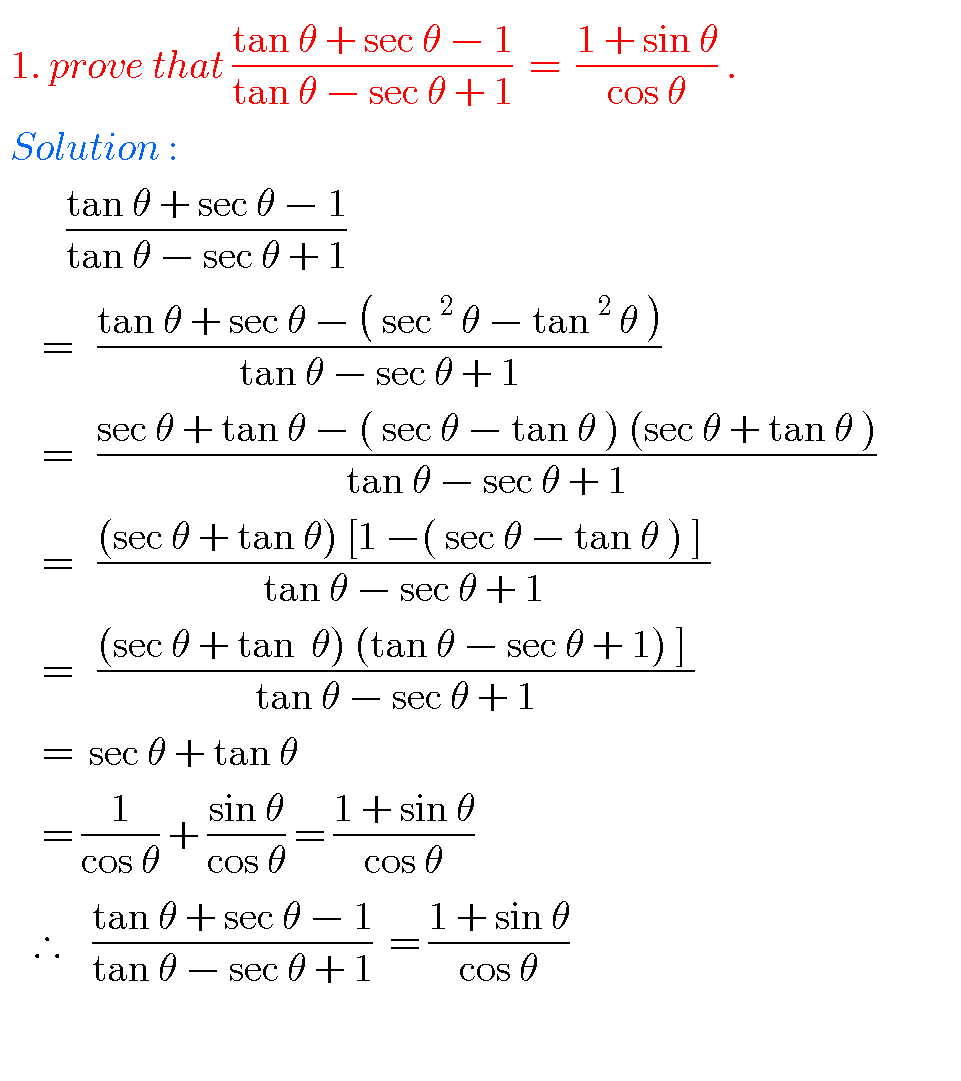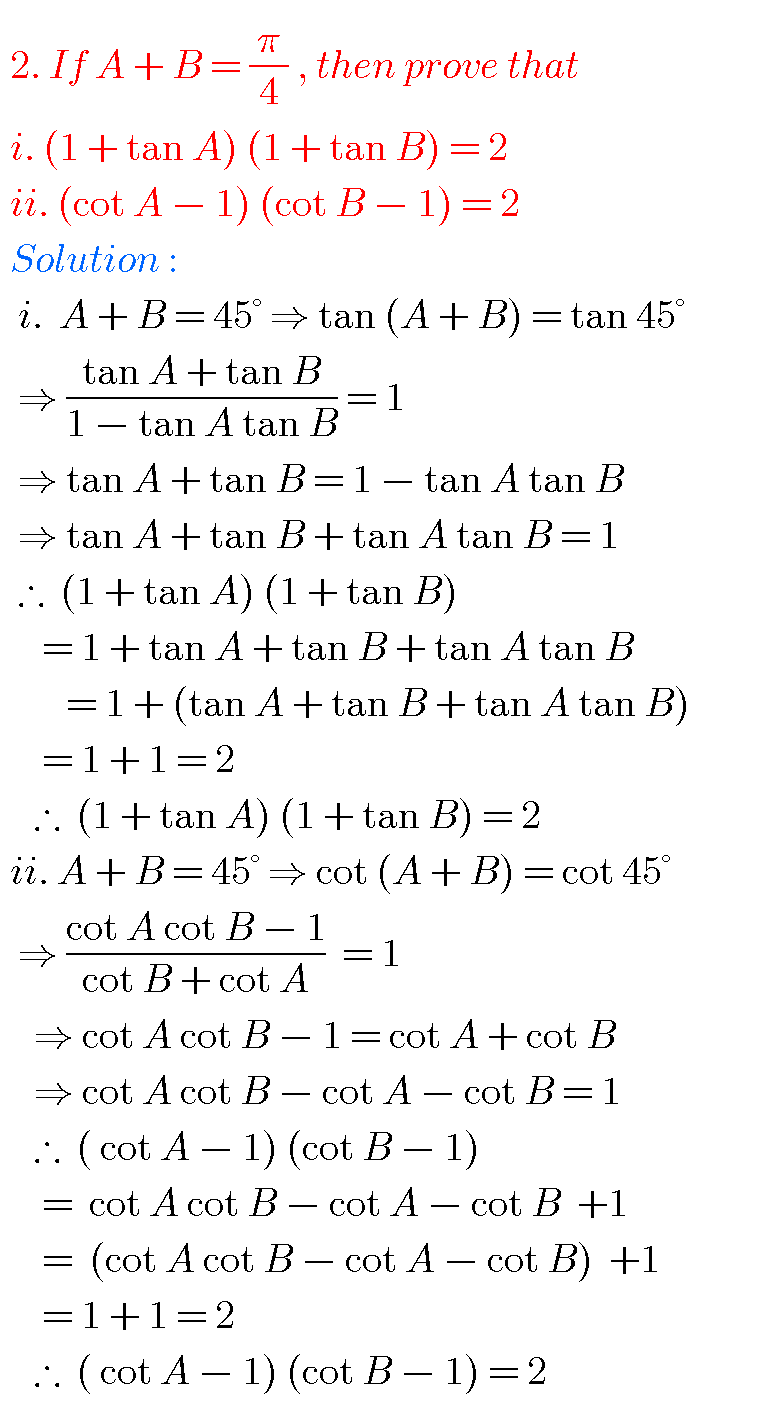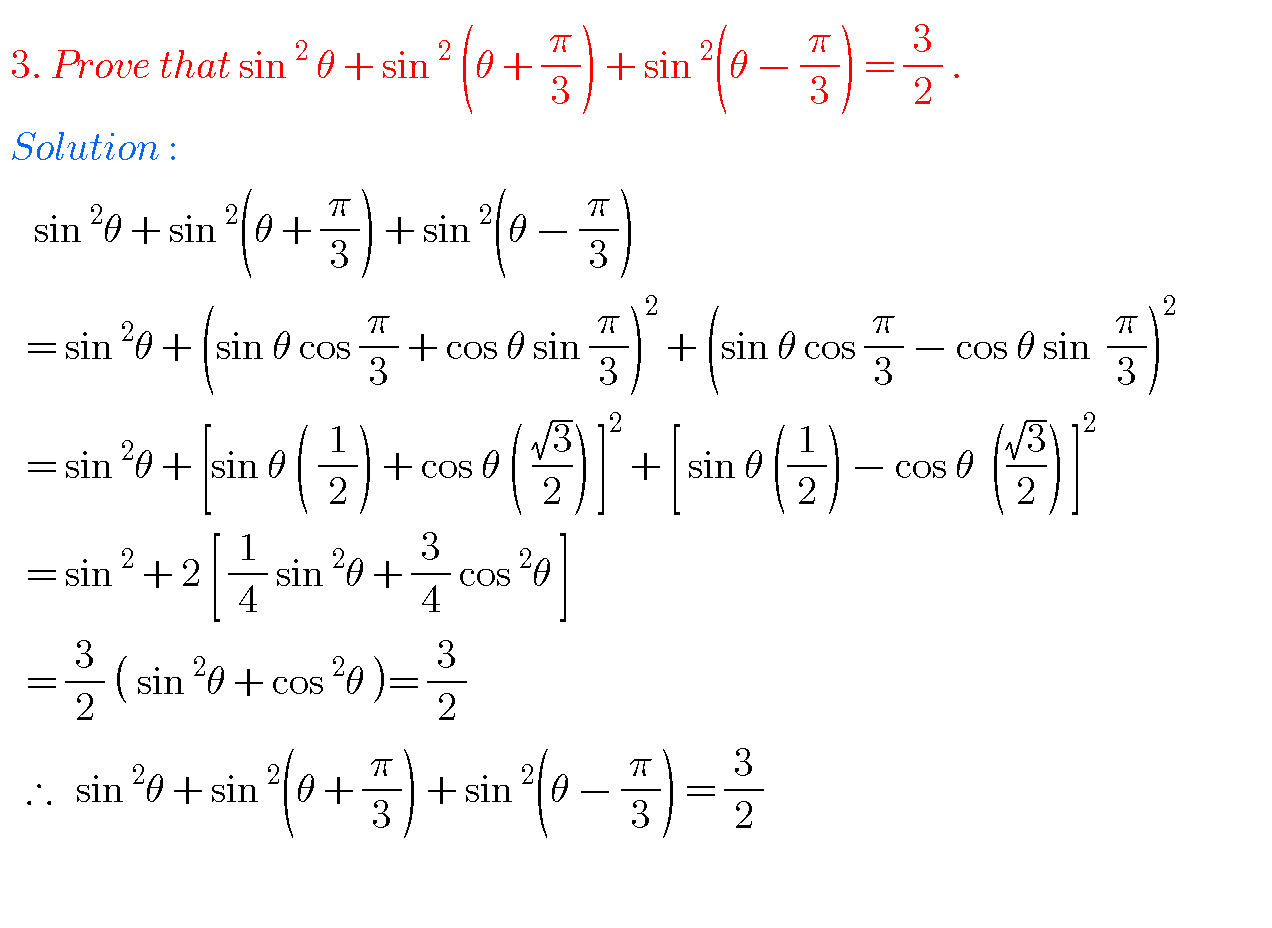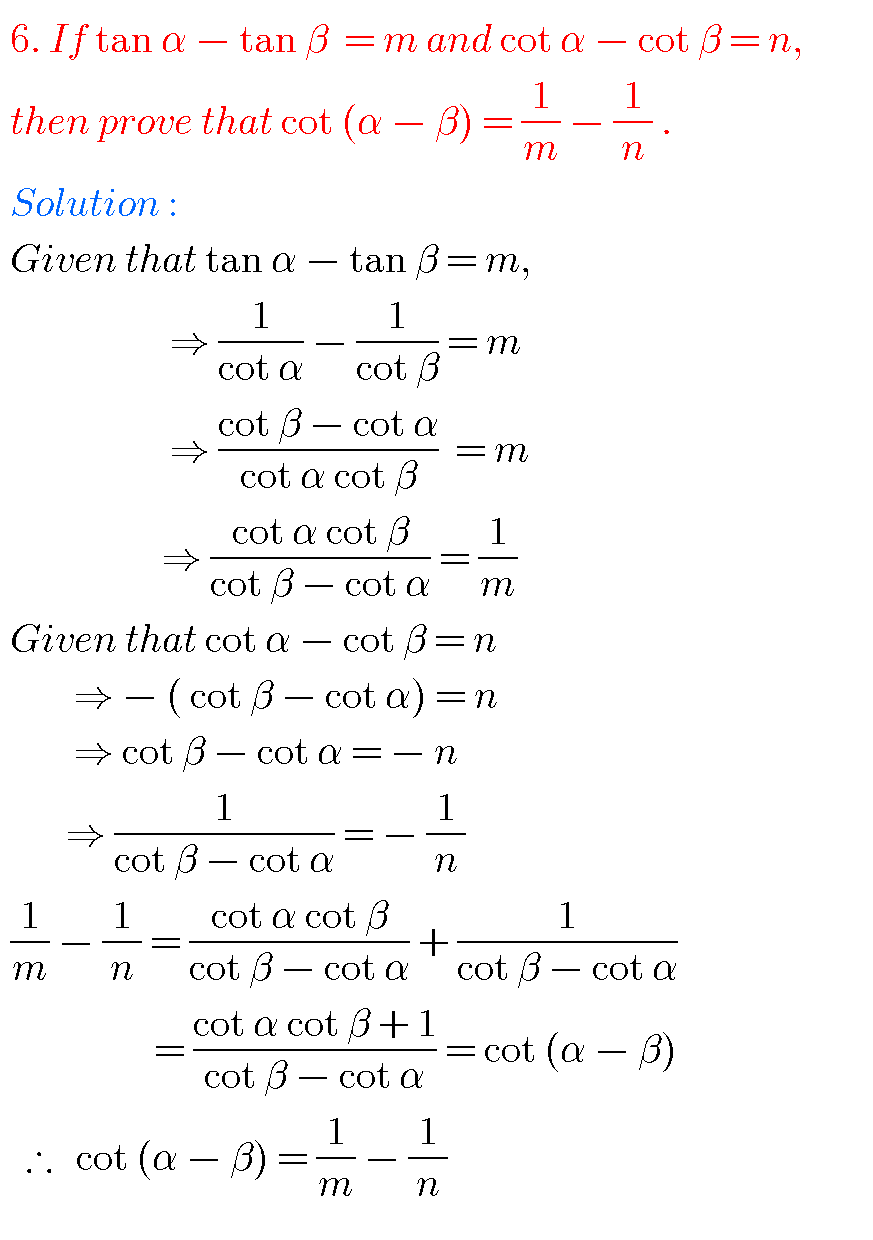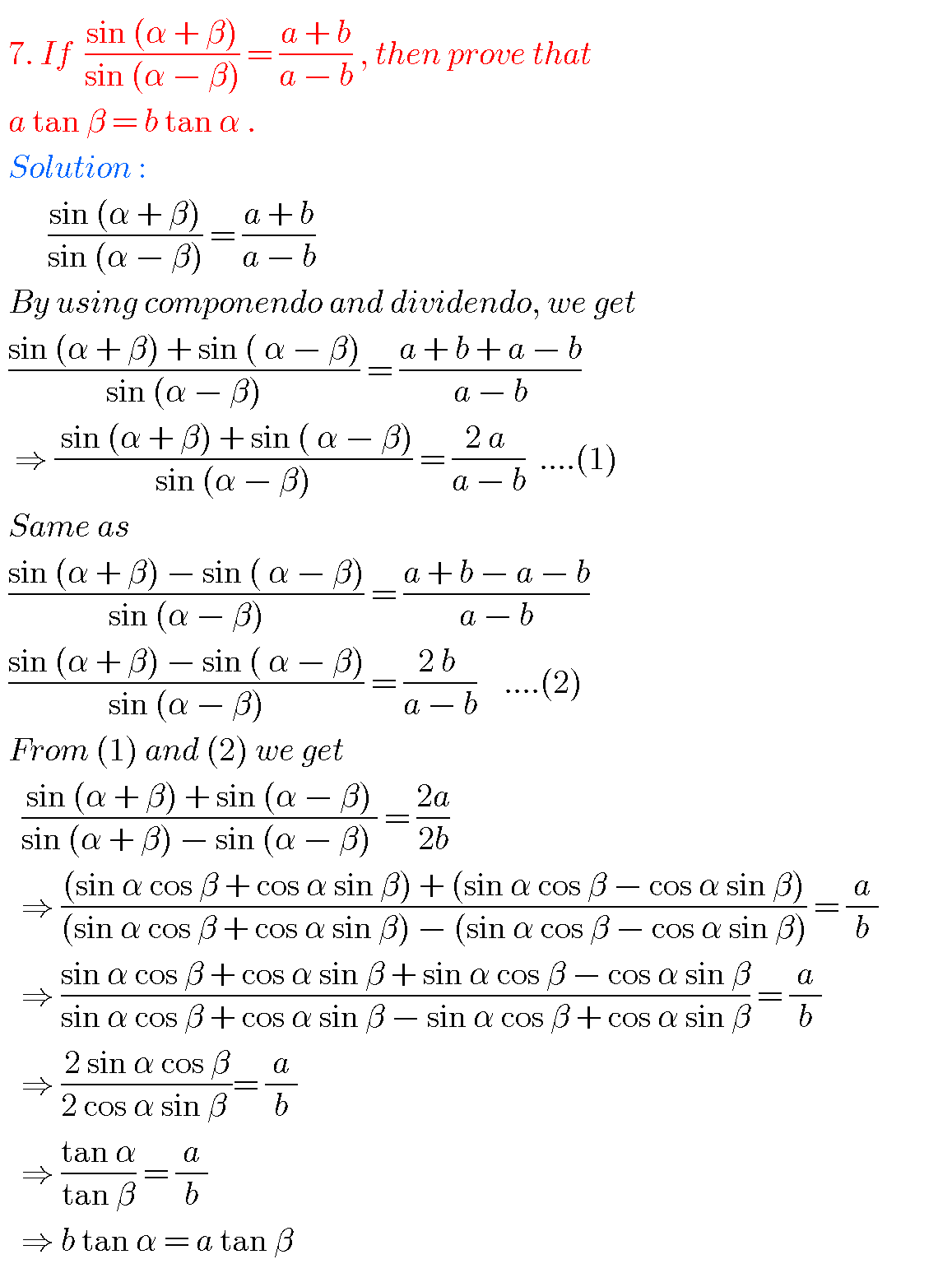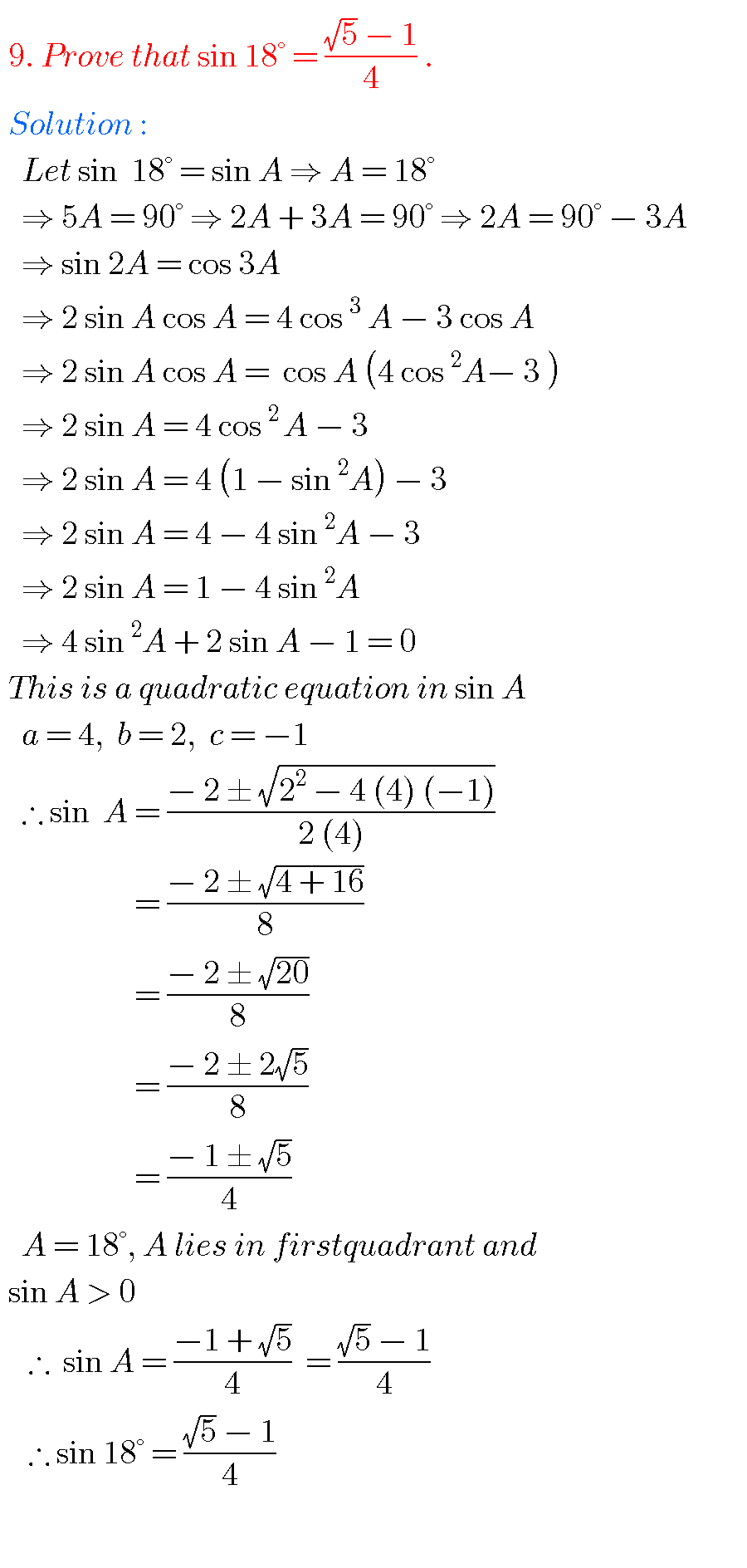# INTERMEDIATE FIRST YEAR 1A PROBLEMS WITH SOLUTIONS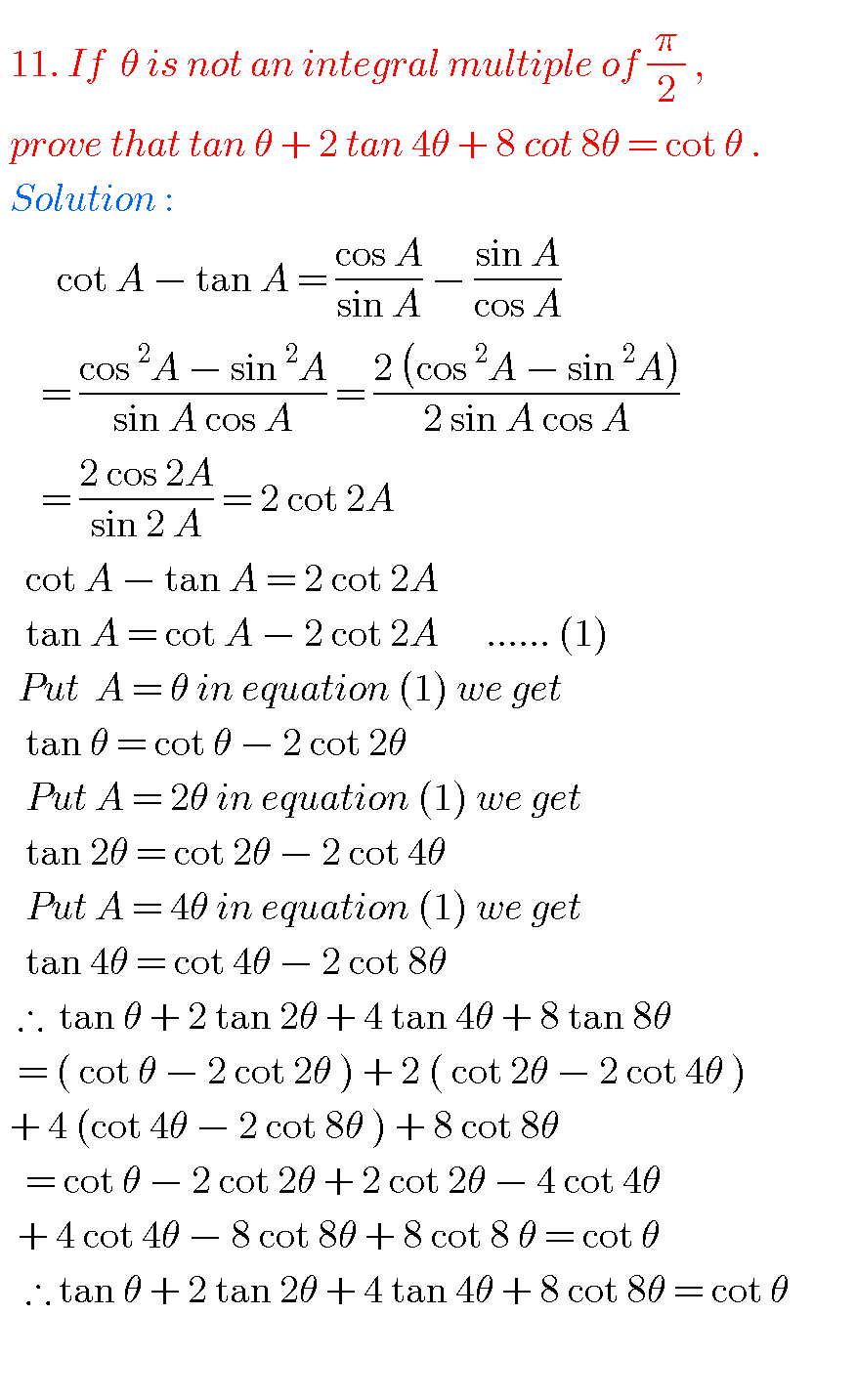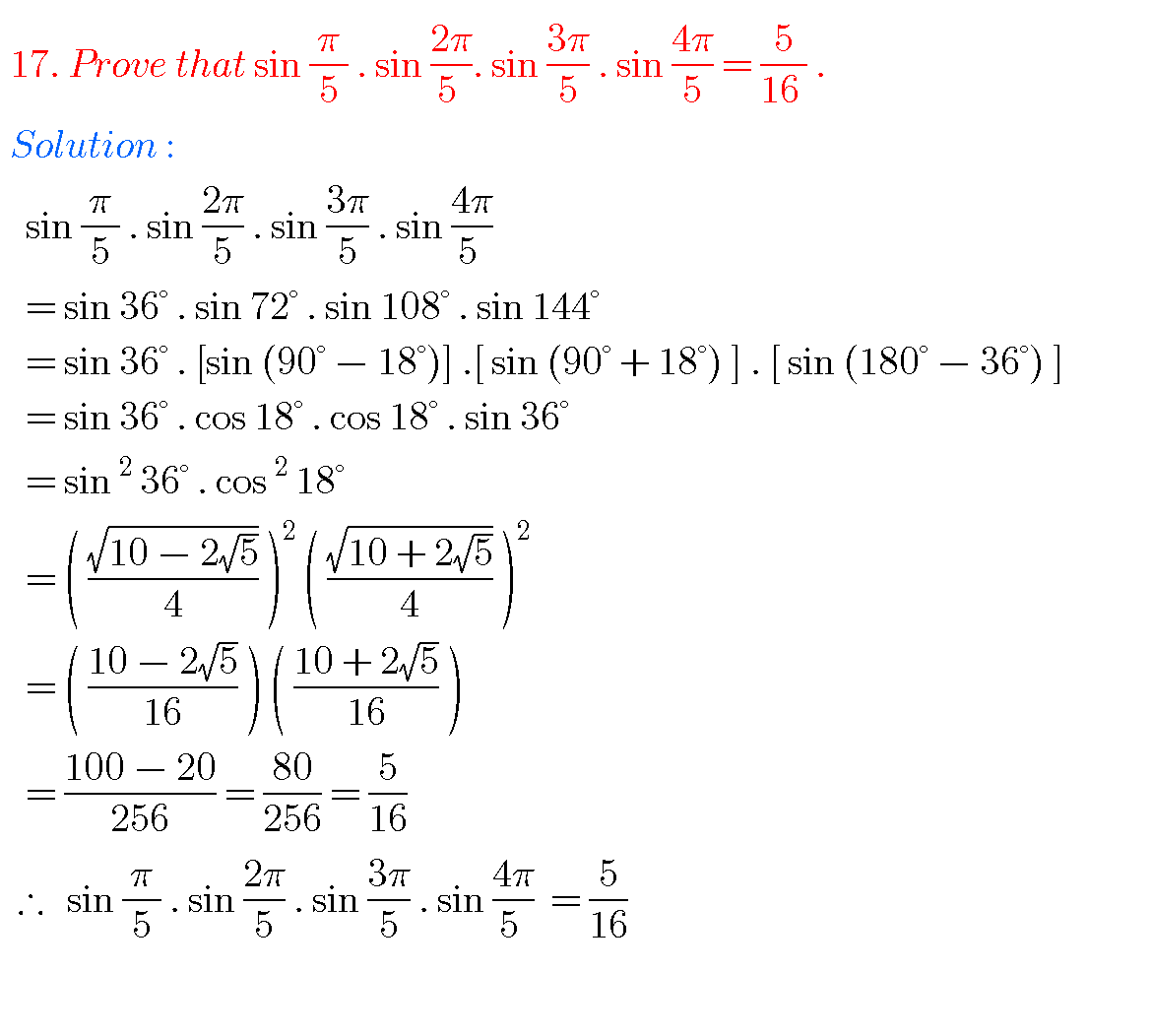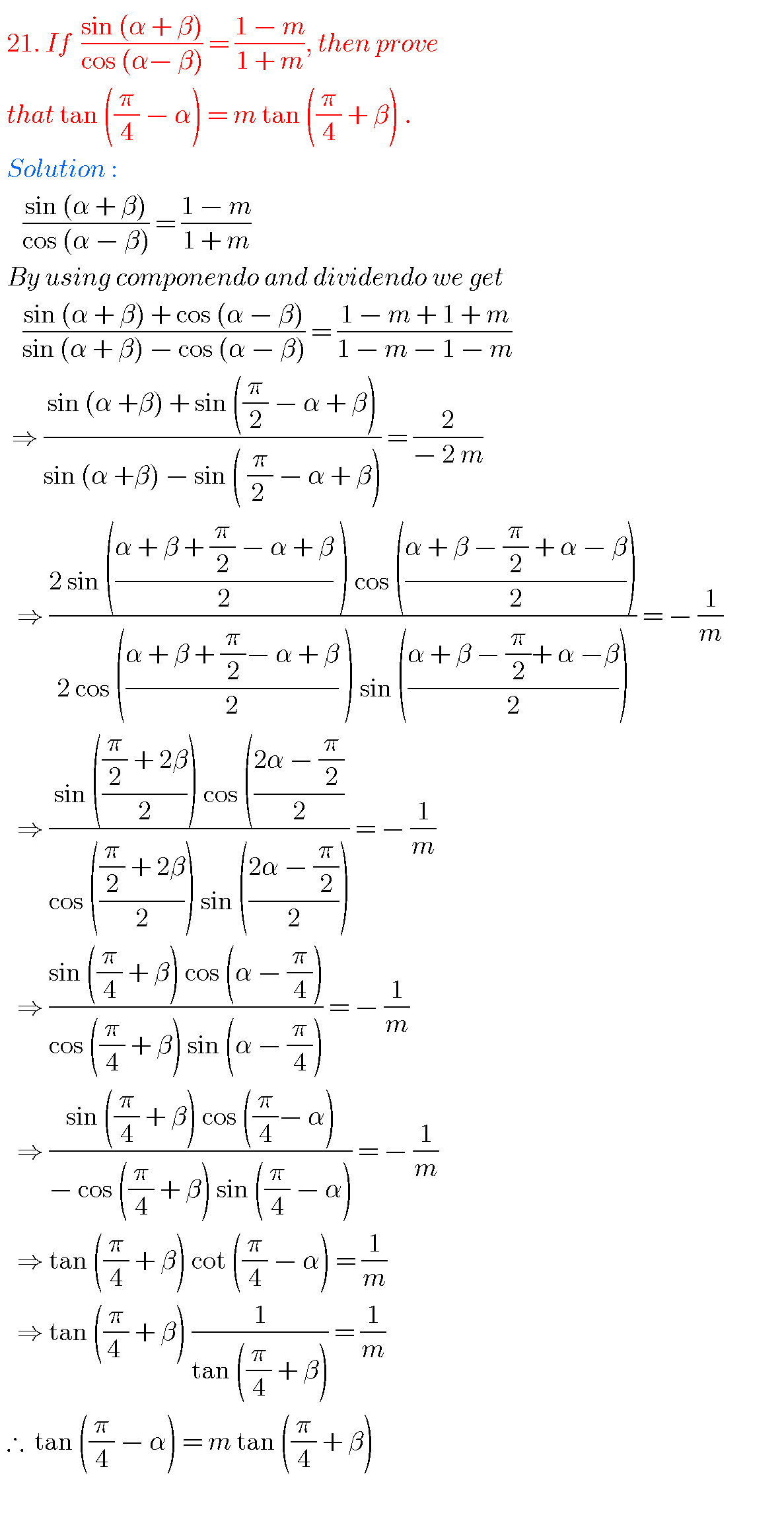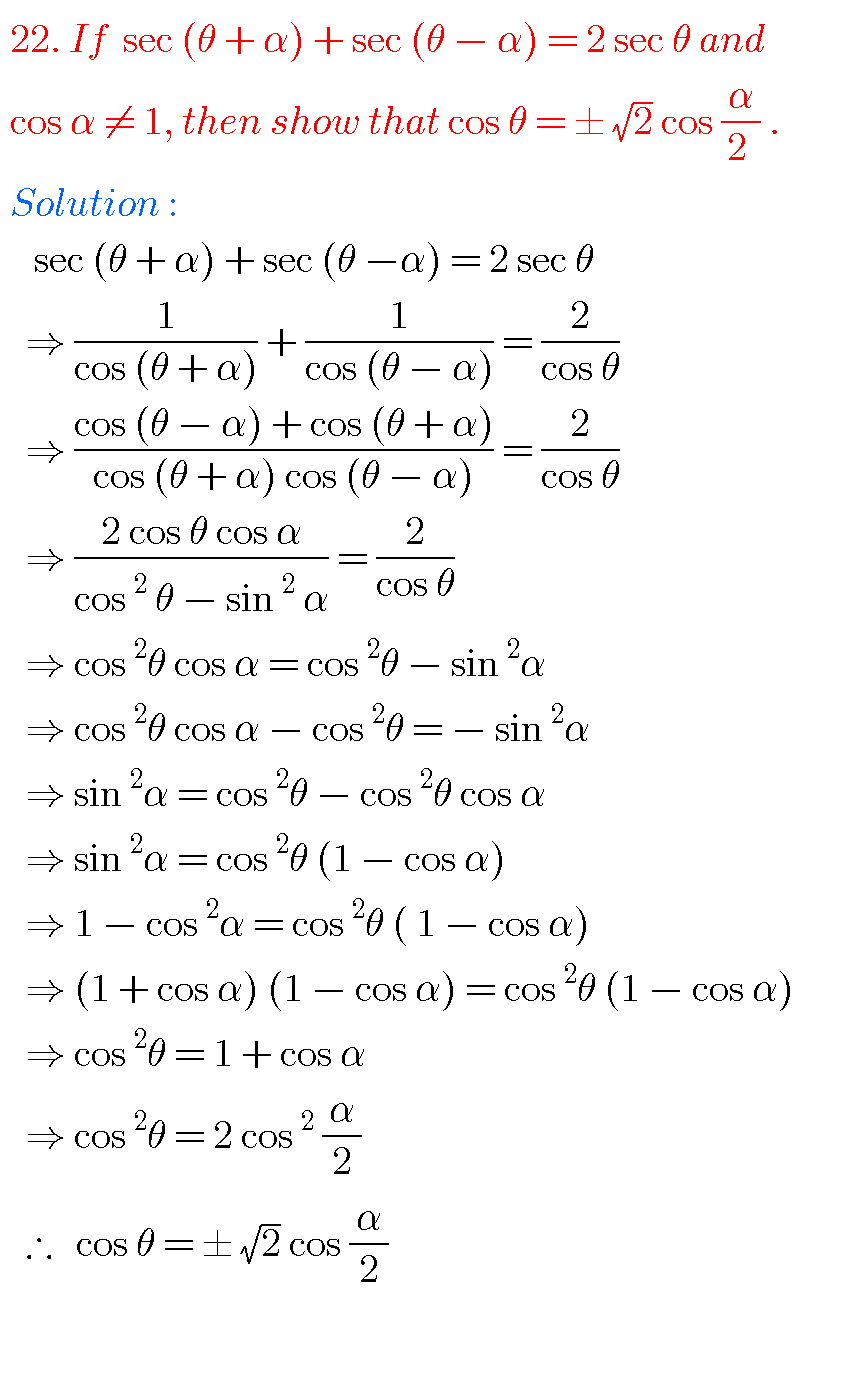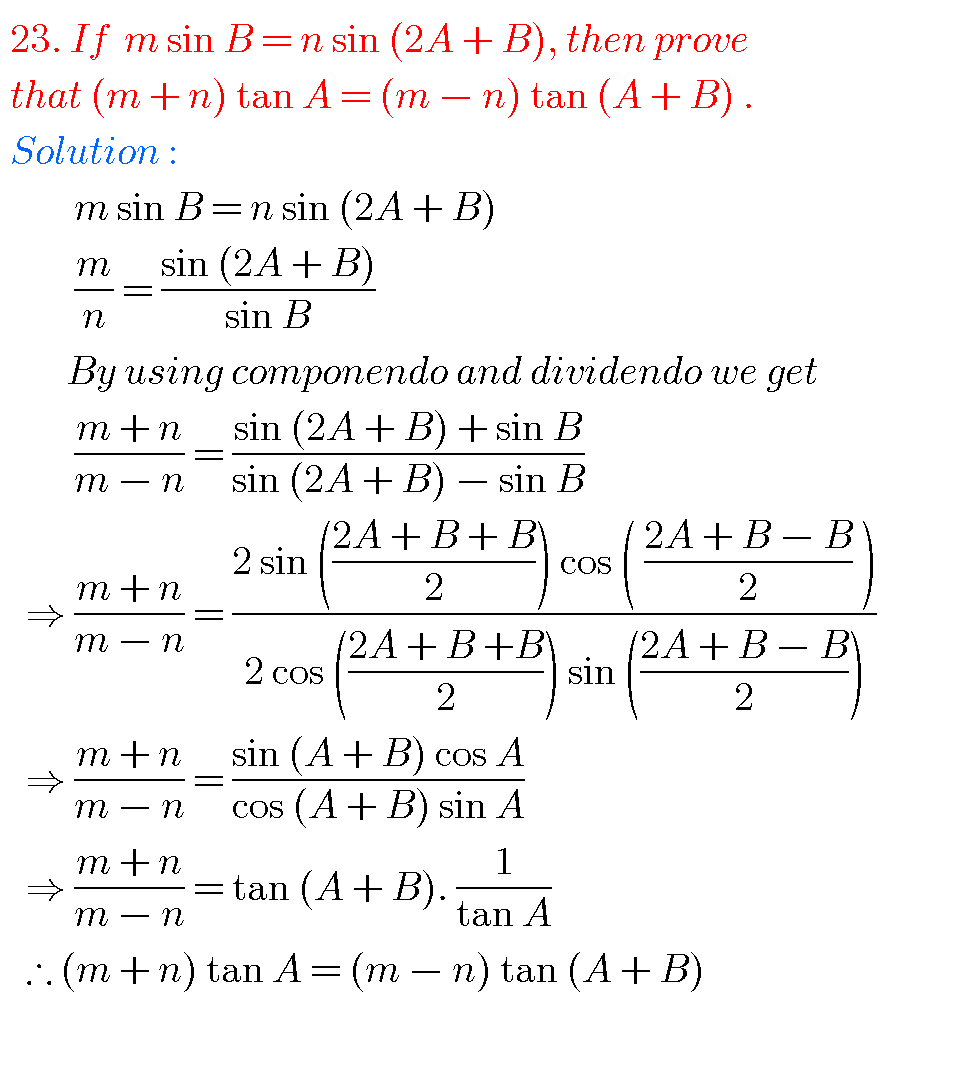## TRIGONOMETRIC RATIOS UPTO TRANSFORMATIONS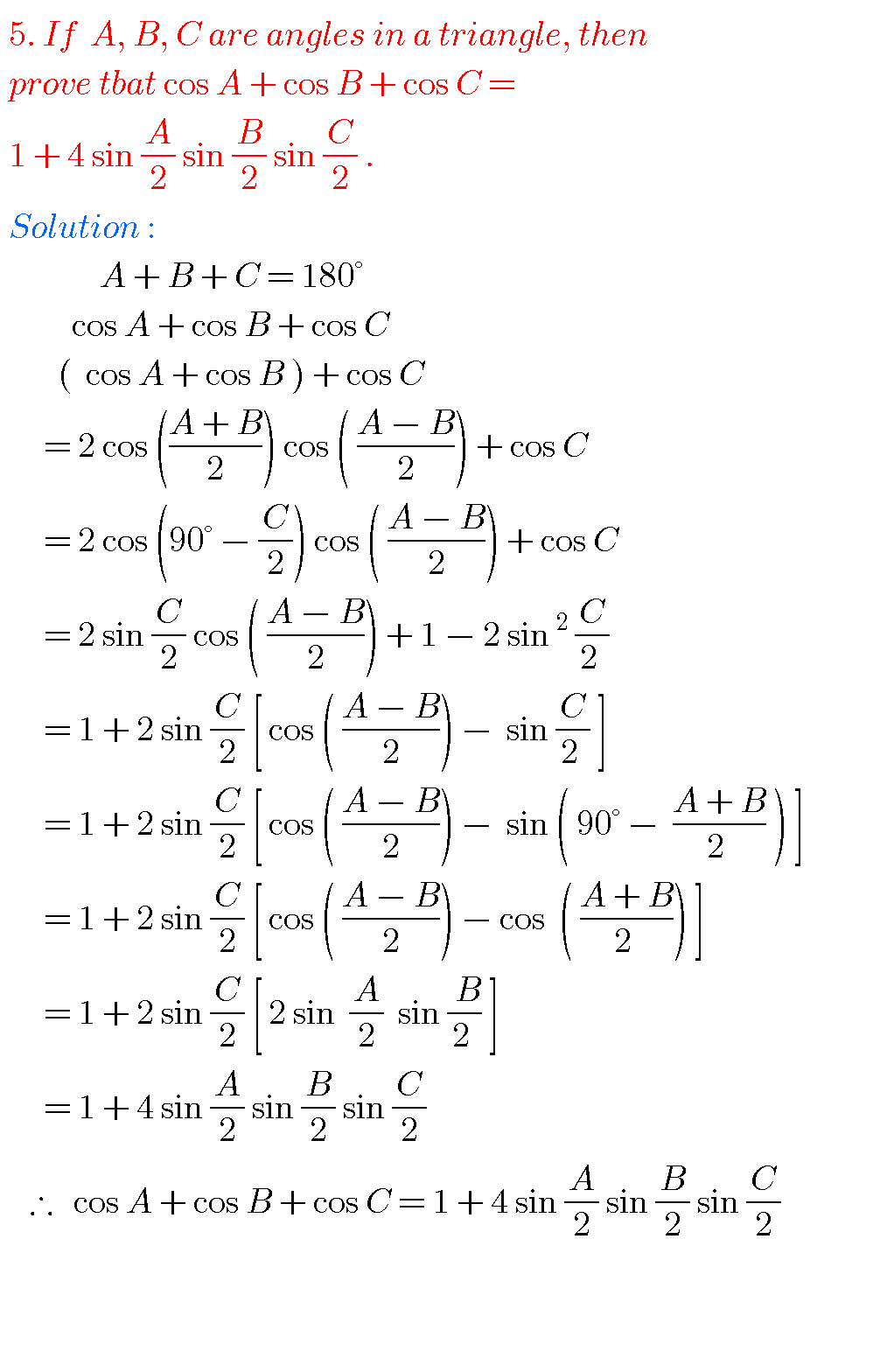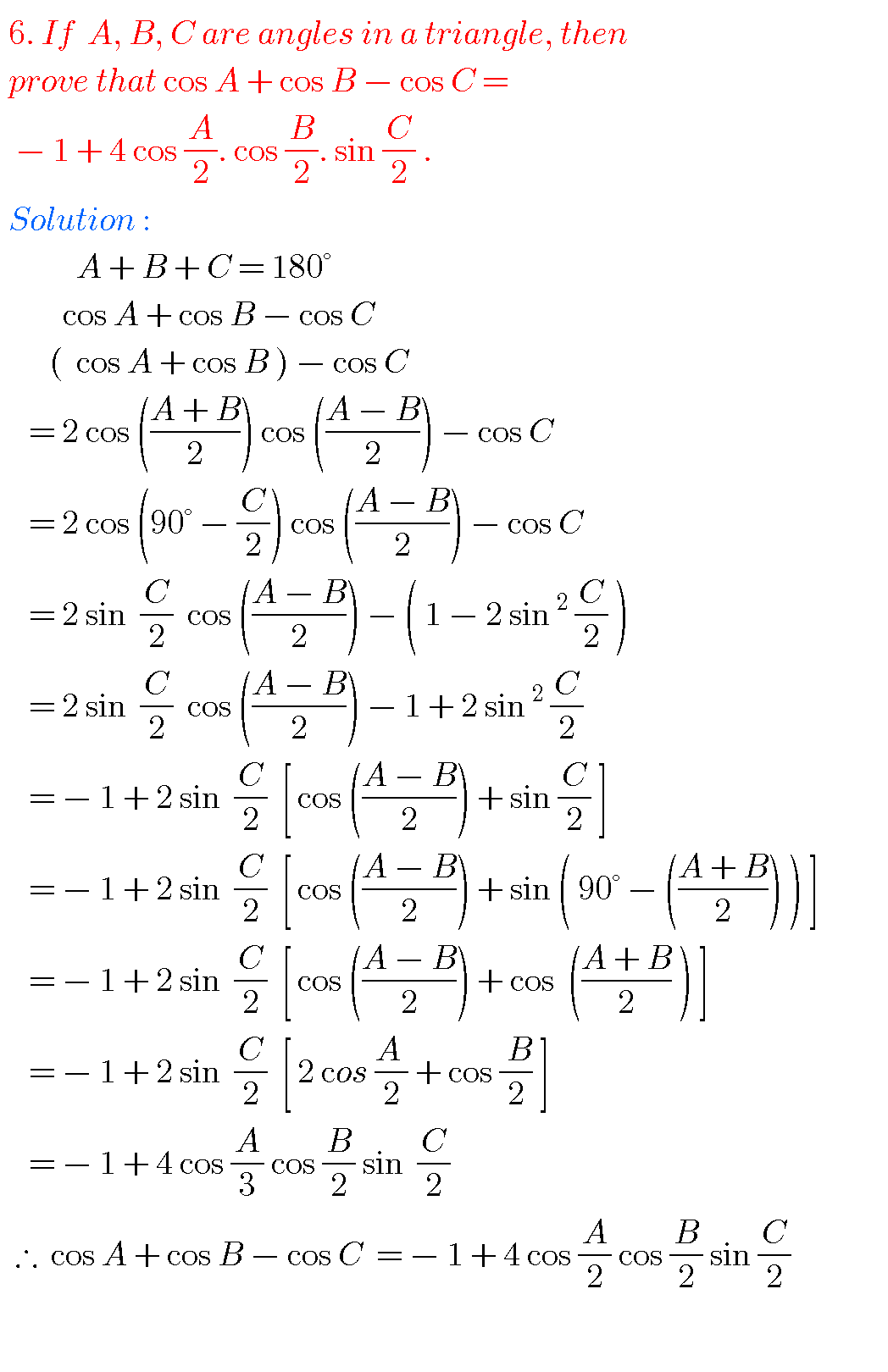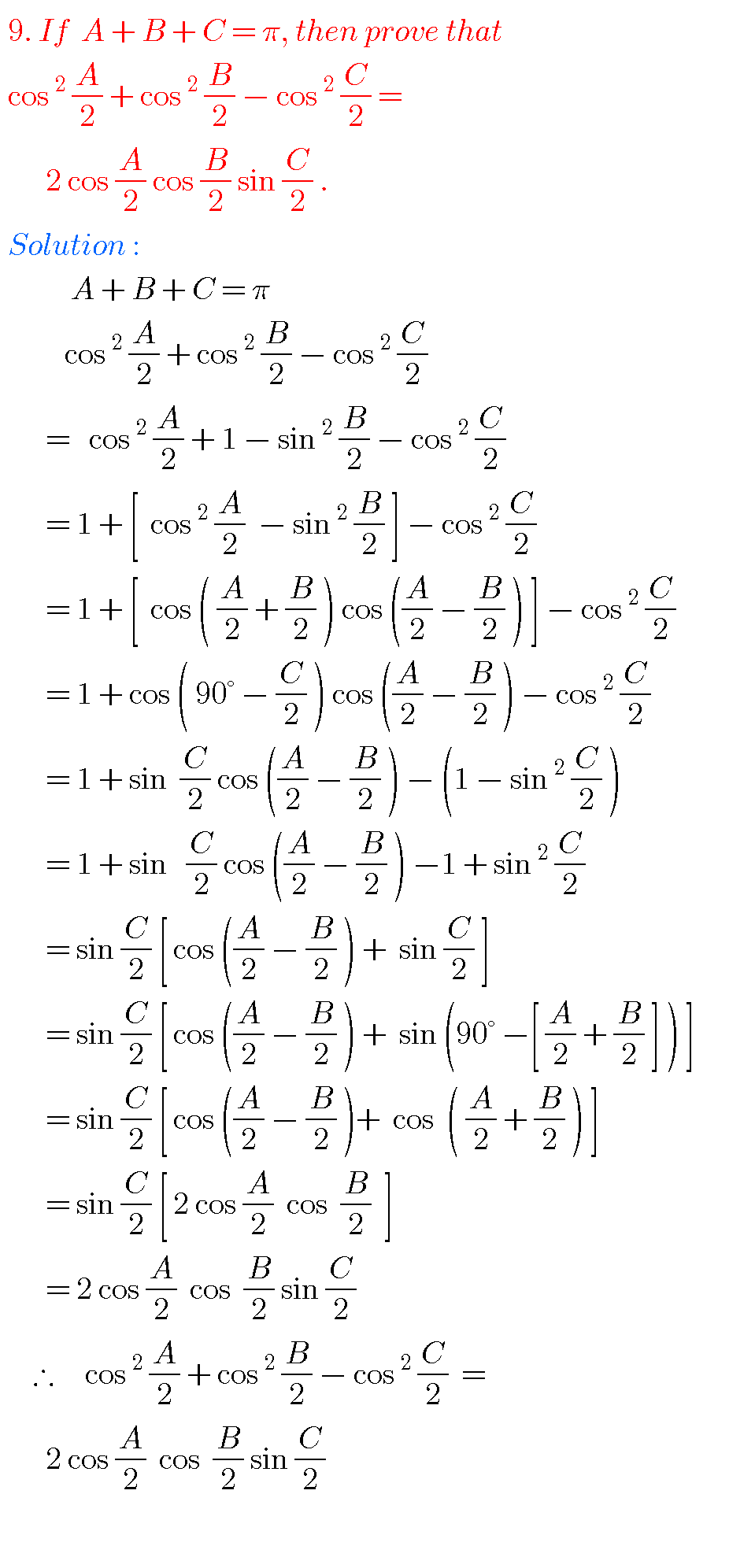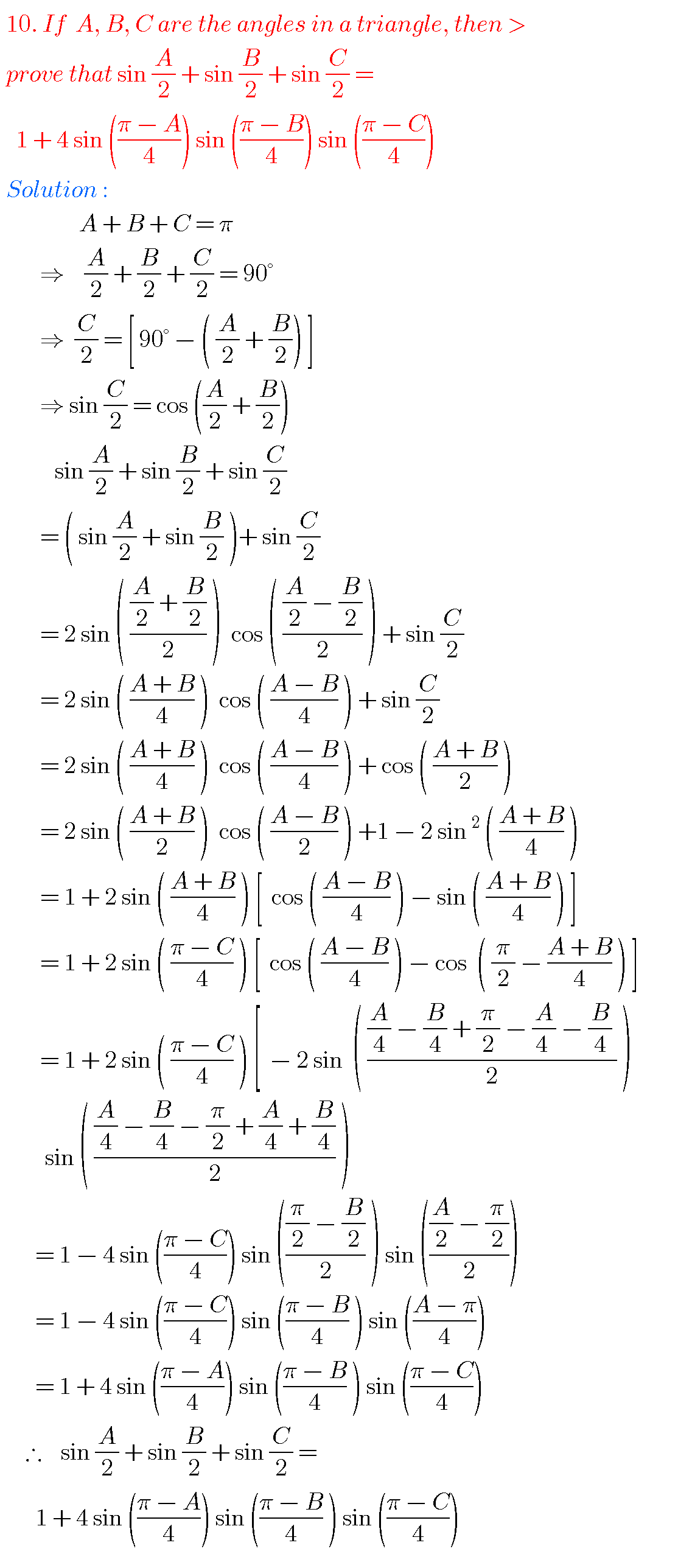## INTERMEDIATE FIRST YEAR 1A PROBLEMS WITH SOLUTIONS

Note : Observe the solutions of trigonometric ratios upto transformations and try them in your own methods.

You can also see

Ncert solutions for maths class 6 th some chapters

Ncert solutions for maths class 7 th some chapters

Ncert solutions for maths class 8 th some chapters

Ncert solutions for class 8 chapter 12

Inter maths 1a solutions

Inter maths trigonometry solutions

You can see solutions for Inter Maths IIB

1. Circle

3. Parabola

4. Ellipse

You can also see solutions for Inter Maths IIA

For examination purpose you can see

Complex numbers

De Moivre’ s Theorem

1.Rohith June 17, 2019 at 11:02 pm - Reply

If cos theta = k , 0 < k < 1 and theta is not a angle in the first quadrant then find the values of sin theta and tan theta in terms of k answer 4 this

•M s dhoni September 18, 2019 at 10:18 pm - Reply

Nice it really helped me a lot

2.polimati greeshma July 25, 2019 at 6:27 am - Reply

u only gave examples

•Sai spl September 7, 2019 at 5:40 am - Reply

Hey it is not examples